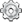# Team:Valencia Biocampus/Demonstration/Diffusion1

(Difference between revisions)
 Revision as of 08:49, 4 October 2013 (view source) (→Proof of a Group Behavior Diffusion Model from a Random Walk Model)← Older edit Latest revision as of 21:16, 4 October 2013 (view source)Lujemomo (Talk | contribs) (→Proof of a Group Behavior Diffusion Model from a Random Walk Model) Line 70: Line 70: Although, there may exist an analytical solution for this differential equation, it has not been found yet as far as we are concerned; that's why we decided to solve it with numerical tools. Although, there may exist an analytical solution for this differential equation, it has not been found yet as far as we are concerned; that's why we decided to solve it with numerical tools.

- ----- + - Okopinska A. (2002) Fokker-Planck equation for bistable potential in the optimized expansion. Physical review E, Volume 65, 062101
+

## Latest revision as of 21:16, 4 October 2013## Proof of a Group Behavior Diffusion Model from a Random Walk Model

In addition to last proof, we will make the assumption that the worm can now move in two dimensions ($x,y$).

As before, time step is $\;q\;$, and space step (either in $x$ or in $y$ coordinate) is $\;h\;$. Each time step $\;q\;$, the worm can move a distance $\;h\;$ either up, down, left or right with probabilities $\;u\;$, $\;d\;$, $\;l\;$ and $\;r\;$, respectively. Nevertheless, now we will consider that this probabilities will be dependent on position, so we must rename them as $\;u(x,y)\;$, $\;d(x,y)\;$, $\;l(x,y)\;$ and $\;r(x,y)\;$. The probability of remaining at the same position is, obviously, $\;1 - u(x,y) - d(x,y) - l(x,y) - r(x,y)\;$. The probability of being in position ($x,y$) at time $\;t\;$, $\;P(x,y,t)\;$ is:

$$P(x,y,t)\;=\;P(x,y,t-q)\;\left(1 - l(x,y) - r(x,y) - u(x,y) - d(x,y)\right) + P(x-h,y,t-q)\;r(x-h,y) + \\ P(x+h,y,t-q)\;l(x+h,y) + P(x,y-h,t-q)\;u(x,y-h) + P(x,y+h,t-q)\;d(x,y+h)$$
Then, expanding each term as a Taylor series, centered in $\;t\;$, $\;x\;$ and $\;y\;$, neglecting the higher order terms $\;O(h^3)\;$ and $\;O(q^2)\;$, respectively:

$$P\;=\;\left(P - q\;\frac{\partial P}{\partial t}\right)\;\left(1 - l - r - u - d\right) + \left(P - q\;\frac{\partial P}{\partial t} - h\;\frac{\partial P}{\partial x} + \frac{h^2}{2}\;\frac{\partial^2 P}{\partial x^2}\right)\;\left(r - h\;\frac{\partial r}{\partial x} + \frac{h^2}{2}\;\frac{\partial^2 r}{\partial x^2}\right) + \\ \left(P - q\;\frac{\partial P}{\partial t} + h\;\frac{\partial P}{\partial x} + \frac{h^2}{2}\;\frac{\partial^2 P}{\partial x^2}\right)\;\left(l + h\;\frac{\partial l}{\partial x} + \frac{h^2}{2}\;\frac{\partial^2 l}{\partial x^2}\right) + \left(P - q\;\frac{\partial P}{\partial t} - h\;\frac{\partial P}{\partial y} + \frac{h^2}{2}\;\frac{\partial^2 P}{\partial y^2}\right)\;\left(u - h\;\frac{\partial u}{\partial y} + \frac{h^2}{2}\;\frac{\partial^2 u}{\partial y^2}\right) + \\ \left(P - q\;\frac{\partial P}{\partial t} + h\;\frac{\partial P}{\partial y} + \frac{h^2}{2}\;\frac{\partial^2 P}{\partial y^2}\right)\;\left(d - h\;\frac{\partial d}{\partial y} + \frac{h^2}{2}\;\frac{\partial^2 d}{\partial y^2}\right) + O(h^3) + O(q^2) + O(h\;q)$$
Which, after operating, results in:

$$q\;\frac{\partial P}{\partial t}\; = \;h\;\left(\frac{\partial l}{\partial x} - \frac{\partial r}{\partial x}\right)\;f + h\;\left(\frac{\partial d}{\partial y} - \frac{\partial u}{\partial y}\right)\;f + \frac{h^2}{2}\left(\frac{\partial^2 r}{\partial x^2} + \frac{\partial^2 l}{\partial x^2}\right)\;f + \frac{h^2}{2}\left(\frac{\partial^2 d}{\partial y^2} + \frac{\partial^2 u}{\partial y^2}\right)\;f + h\;\left(l - r\right)\;\frac{\partial f}{\partial x} + h\;\left(d - u\right)\;\frac{\partial f}{\partial y} + \\ h^2\;\left(\frac{\partial l}{\partial x} + \frac{\partial r}{\partial x}\right)\;\frac{\partial f}{\partial x} + h^2\;\left(\frac{\partial d}{\partial y} + \frac{\partial u}{\partial y}\right)\;\frac{\partial f}{\partial y} + \frac{h^2}{2}\;\left(l + r\right)\;\frac{\partial^2 f}{\partial x^2} + \frac{h^2}{2}\;\left(d + u\right)\;\frac{\partial^2 f}{\partial y^2} + O(h^3) + O(q^2) + O(h\;q)$$
Now we define:

$$\nu_x\;=\;\lim\limits_{h,\;q,\;\beta_x\rightarrow 0}\frac{\beta_x\;h}{q}$$ $$\nu_y\;=\;\lim\limits_{h,\;q,\;\beta_y\rightarrow 0}\frac{\beta_y\;h}{q}$$ $$a_{x,x}\;=\;\lim\limits_{h,\;q\rightarrow 0}\frac{\alpha_x\;h^2}{2q}$$ $$a_{y,y}\;=\;\lim\limits_{h,\;q\rightarrow 0}\frac{\alpha_y\;h^2}{2q}$$
Where $\;\alpha_x \;=\; r + l\;$, $\;\alpha_y \;=\; u + d\;$, $\;\beta_x \;=\; r - l\;$ and $\;\beta_y \;=\; u - d\;$.

We now take the equation, divide it by $\;q\;$ and take the limit as $\;h,q,\beta_x,\beta_y\rightarrow 0\;$ such that $\;\beta_x h/q\;$, $\;\beta_y h/q\;$, $\;\alpha_x h^2/2q\;$ and $\;\alpha_y h^2/2q\;$ all tend to a constant, obtaining:

$$\frac{\partial f}{\partial t}\;=\;\frac{\partial^2 f a_{x,x}}{\partial x^2} + \frac{\partial^2 f a_{y,y}}{\partial y^2} - \frac{\partial f \nu_x}{\partial x} - \frac{\partial f \nu_y}{\partial y}$$
Or, what is the same:

$$\frac{\partial f}{\partial t}\;=\;\nabla·\left(\nabla\left(\underline{\underline{D}} f\right)\right) - \nabla·\left(\underline{\nu} f\right)$$
With:

$$\underline{\underline{D}} \; = \begin{bmatrix} a_{x,x}(x,y) & 0\\ 0 & a_{y,y}(x,y)\\ \end{bmatrix} \;\;\;\;\;\;\;\;\;\;\;\; \underline{\nu} \; = \begin{bmatrix} \nu_x(x,y)\\ \nu_y(x,y)\\ \end{bmatrix}$$
Nevertheless, in our case of study, we dealed with $\;D\;$ as a constant, so $\;a_{x,x}(x,y)\;=\;a_{y,y}(x,y)\;=\;D\;$, so we can tanke it out from the Laplacian, obtaining what we were waiting anxiously:

$$\frac{\partial f}{\partial t}\;=\;D\;\nabla^2 f - \nabla·\left(\underline{\nu} f\right)$$
Although, there may exist an analytical solution for this differential equation, it has not been found yet as far as we are concerned; that's why we decided to solve it with numerical tools.